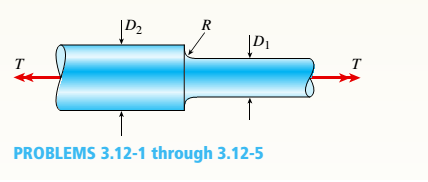# A stepped shaft (see figure) has diameter D 2 = 1.5 in, and a full quarter-circular fillet. The allowable shear stress is 15,000 psi and the load T = 4800 1b-in. What is the smallest permissible diameter D 1 ?### Mechanics of Materials (MindTap Co...

9th Edition
Barry J. Goodno + 1 other
Publisher: Cengage Learning
ISBN: 9781337093347### Mechanics of Materials (MindTap Co...

9th Edition
Barry J. Goodno + 1 other
Publisher: Cengage Learning
ISBN: 9781337093347
Chapter 3, Problem 3.12.5P
Textbook Problem

## A stepped shaft (see figure) has diameter D2= 1.5 in, and a full quarter-circular fillet. The allowable shear stress is 15,000 psi and the load T = 4800 1b-in.What is the smallest permissible diameter D1?Expert Solution
To determine

The minimum value of smaller diameter is 1.31in.

### Explanation of Solution

Given Information:

The larger diameter of the shaft is 1.5in, allowable shear stress is 15000psi, and torque applied on the shaft is 4800lbin.

Write the expression for the radius of the full quarter circular fillet.

R=D2D12....... (I)

Write the expression for the ratio of the diameters of the shaft.

n=D2D1....... (II)

Here, the larger diameter of the shaft is D2, and smaller diameter of the shaft is D1.

Write the expression for the ratio of fillet radius to the smaller diameter of the shaft.

r=RD1....... (III)

Here, the fillet radius is R.

Write the expression for the shear stress on the shaft.

τmax=K16Tπd3....... (IV)

Here, the torque on the shaft is T, and stress concentration factor of the shaft is K.

Calculation:

Substitute 1.5infor D2in Equation (I).

R=1.5inD12=0.75inD12

Substitute 1.5infor D2in Equation (II).

n=1.5inD1

Substitute 0.75inD12for Rin Equation (III).

r=0.75inD12D1=0

### Want to see the full answer?

Check out a sample textbook solution.See solution

### Want to see this answer and more?

Bartleby provides explanations to thousands of textbook problems written by our experts, many with advanced degrees!

See solution